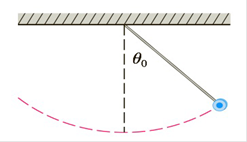Chapter 7.7, Problem 42E

Chapter
Section
Textbook Problem

# The figure shows a pendulum with length L that makes a maximum angle θ 0 with the vertical. Using Newton’s Second Law, it can be shown that the period T (the time for one complete swing) is given by T = 4 L g ∫ 0 π / 2 d x 1 − k 2 sin 2 x where k = sin ( 1 2 θ 0 ) and g is the acceleration due to gravity. If L = 1  m and θ 0 = 42 0 , use Simpson’s Rule with n = 10 to find the period.To determine

To Find: The time period using Simpson’s Rule for n=10

Explanation

Calculations: Applying Simpson’s Rule for n=10, we get

T=419.8π203[f(0)+4(f(π20)+f(3π20)+f(5π20)++f(7π20)+f(9π20))+2(f(2π20)+f(4π20)+f

### Still sussing out bartleby?

Check out a sample textbook solution.

See a sample solution

#### The Solution to Your Study Problems

Bartleby provides explanations to thousands of textbook problems written by our experts, many with advanced degrees!

Get Started

#### What is wrong with the equation? /3sectand=sec]/3=3

Single Variable Calculus: Early Transcendentals, Volume I

#### In Exercises 516, evaluate the given quantity. log22

Finite Mathematics and Applied Calculus (MindTap Course List)

#### True or False: f(x) = x2 is decreasing for 10 x 1.

Study Guide for Stewart's Single Variable Calculus: Early Transcendentals, 8th